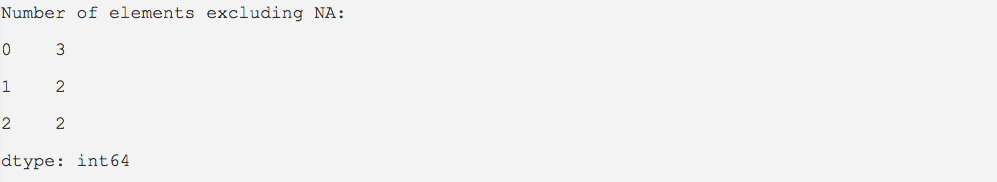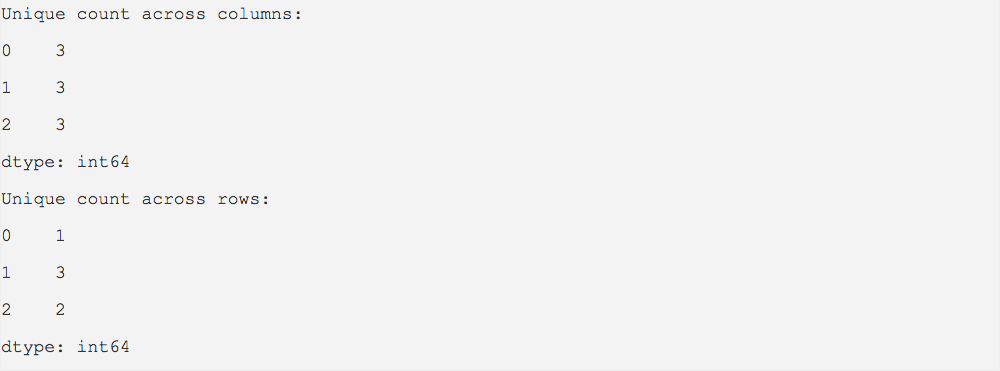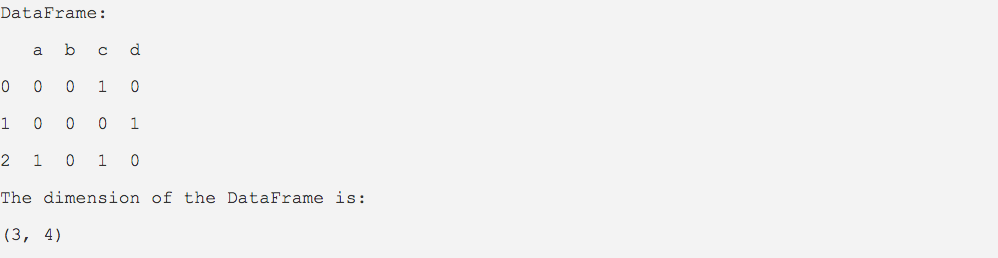# Count Functions And Related Attributes Of Pandas DataFrame

## Overview:

• The count() method of DataFrame class returns the number of non-NA elements present in each row or each column of a DataFrame instance.
• The nunique() method counts the number of unique values in a row or a column of DataFrame. Using dropna parameter the NA values can be ignored.
• The shape attribute returns the dimension of a DataFrame instance.

## Example for count() method:

 import pandas as pd   dataValues = [(5, 10, None),               (15, 20, 25),               (35, None, 40)]; dataFrame = pd.DataFrame(data = dataValues);   print("Number of elements excluding NA:"); countValues = dataFrame.count(); print(countValues);

## Output:

Note that the return type of the count() method is a series. Also the first column in the output is the index of the series. The second column in the output is the counts corresponding to the columns present in the DataFrame.## Example for nunique() method:

 import pandas as pd   dataValues = [(5,5,5),               (1,2,3),               (7,0,7)]; dataFrame       = pd.DataFrame(data=dataValues); uniqueCounts    = dataFrame.nunique(); print("Unique count across columns:") print(uniqueCounts);   uniqueCounts    = dataFrame.nunique(axis=1); print("Unique count across rows:") print(uniqueCounts);

## Output:## Example for DataFrame.shape:

 import pandas as pd   values = [(0,0,1,0),           (0,0,0,1),           (1,0,1,0)] dataFrame = pd.DataFrame(data = values,columns=('a','b','c','d'));   print("DataFrame:") print(dataFrame)   print("The dimension of the DataFrame is:") print(dataFrame.shape)

## Output: